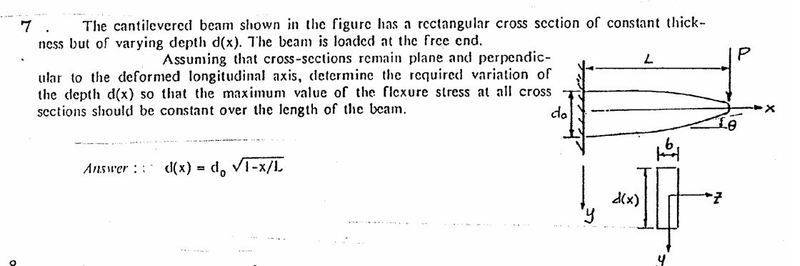# Transverse loading with varing cross sectioni know that

τ=VQ/(Ib)

now im looking for the maximum stress so i will find that at the point at the centre of the rectangle

Q=A*$$\bar{y}$$
A=0.5*d*b
$$\bar{y}$$=0.25*d
===> Q=0.125d2b

I=(b*d3)/12

τ=VQ/(Ib)
τ=V*0.125d2b*12/((b2*d3)

τ=1.5V/(bd)

now d changes as a function of X

i know that τ is constant from x=0 to x=L
but V is constant throughout and so is b, so how can this be?

i tried making a differential equation where i know d(0)=do and d(L)=0 using dτ/dx=0 but really didnt manage

## Answers and Replies

PhanthomJay
Science Advisor
Homework Helper
Gold Member
You are calculating max shear stress, but the problem is asking for the variation in d such that max flexure stress (bending stress) under the transverse load P is constant throughout the beam. Try again using the formula for max bending stress.

am i meant to be using -y*(M/I)

i know the following

Ymax=d/2
M=PL-Px=P(L-x)
I=(bd3)/12

therefore

max stress=(d/2)*P(L-x)/((bd3)/12)

and i get
6P(L-x)/(bd2)

from here i got it thanks